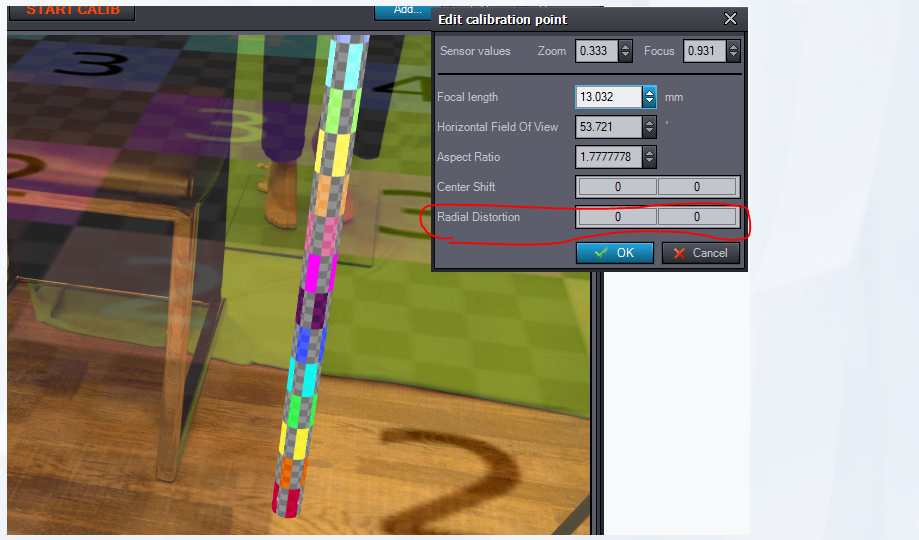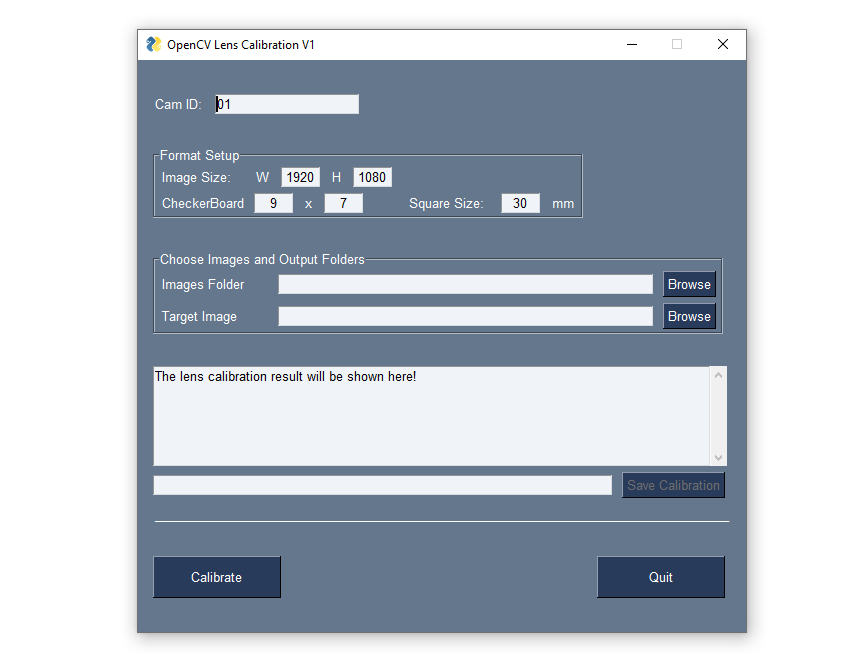Studio Operators Camera Tracking

Hi Axy Team,

I wrote an OpenCV lens calibration app recently...

https://github.com/graphicsvending/OpenCV_LensCalibration

and it generated lens calibration Json file with distortion coefficients (K1,K2,P1,P2,K3)

Does the math formula works for the radial distortion input on the edit calibration point?

x-distorted = x(1 + k1*r2 + k2*r4+ k3*r6)

y-distorted= y(1 + k1*r2 + k2*r4 + k3*r6)

x, y — undistorted pixels that are in image coordinate system.

k1, k2, k3 — radial distortion coefficients of the lens.

reference from:xdistorted=x(1+k1r2+k2r4+k3r6)ydistorted=y(1+k1r2+k2r4+k3r6zeketanAximmetry
7/30/2021 8:43:21 AM
-

Hi,

Yes we use this formula. (assuming by r2 you meant r^2 and so on)

Just be careful, because the coefficients depends on how you interpret x and y. (for example are they in pixel or in screen ratio)

We interpret as in pixel.

Radial Distortion is K1 and K2. The rest (P1,P2 and K3) is not used since they contributed too little based on our tests, but we might reconsider it.

The best if you do your calculation in way that your force P1, P2 and K3 to zero. (which can be done in openCV)

Our Center Shift is in image ratio from the center. So if you use the default openCV output (which is in pixel from the top left corner) then you have to convert that.

cx= cx / width - 0.5

cy= cy / height - 0.5

Best Regards